Next: Index Assistant Functions Up: Software Oriented Approaches Previous: Software Oriented Approaches

### Complete IndexingIn [HM89] Timothy Hickey and Shyam Mudambi present several indexing techniques based on the WAM. The first one (complete indexing) uses global information (like modes) to perform indexing.

First of all the program is transformed, creating new special code for each mode that might occur for a procedure call.

As an example we look at the following program:

```
1. top :- p([1,2,3,4],X), write(X).
2. p([],0).
3. p([X|Y],N) :- p(Y,M), N is M+1.```

p is only called with a constant argument in the first position and a variable in the second. The new code for the procedure p is specialized for this mode. It is represented in the procedure p_cd. If we assume that the program p is also called with other modes, the compiler will produce other specialized procedures for these modes.

The transformed source program is:

```
1. top :- p_cd([1,2,3,4],X), write_c(X).
2. p_cd([],0).
3. p_cd([X|Y],N) :- p_cd(Y,M), N is M+1.```

Then the clauses are transformed into a normal form:
1. p_ccdd(
2.
3.
.

Where:
The generated indexing code is in some sense also a three level indexing (c.f. section 4) of the following form: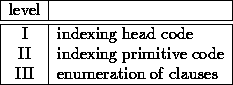The first one is a sequential indexing on the firstc-mode arguments. This is done by unifying the known structure of these arguments and indexing inner different possibilities with a new index-instruction called g_switch reg table. This new instruction assumes that the argument register reg contains a ground term, and switches to the appropriate location after a hash-table look up in table.

The indexing primitive code contains a set of new WAM branch instructions (e.g. if_gt, if_eq, if_le), so control jumps to a given label based e.g. on arithmetical comparisons.

The indexing bodies are compiled with the standard WAM techniques.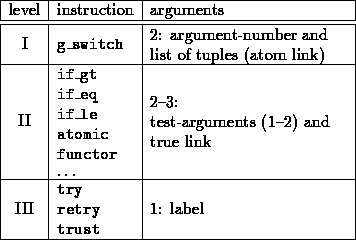Example:

```
1. merge_ccd(L,[],L).
2. merge_ccd([],[B|Bs],[B|Bs]).
3. merge_ccd([A|As],[B|Bs],[A|Cs]) :- A < B,
merge_ccd(As,[B|Bs],Cs).
4. merge_ccd([A|As],[B|Bs],[B|Cs]) :- A >= B,
merge_ccd([A|As],Bs,Cs).```
Normal form:
```
1. merge_ccd(L,[],X1) :- X1=L.
2. merge_ccd([],[B|Bs],X1) :- X1=[B|Bs].
3. merge_ccd([A|As],[B|Bs],X1) :- A < B, X1=[A|Cs],
merge_ccd(As,[B|Bs],Cs).
4. merge_ccd([A|As],[B|Bs],X1) :- A >= B, X1=[B|Cs],
merge_ccd([A|As],Bs,Cs).```
Index tree:
Next: Index Assistant Functions Up: Software Oriented Approaches Previous: Software Oriented Approaches

Michael Sintek - sintek@dfki.uni-kl.de